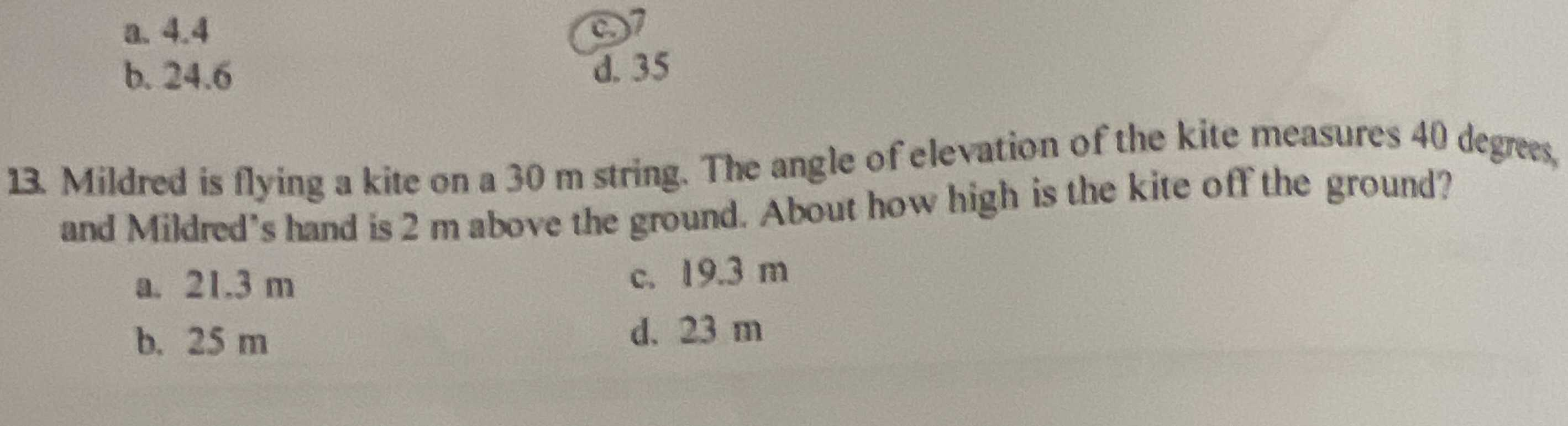### Still have math questions?

Trigonometry
Question13. Mildred is flying a kite on a $$30 m$$ string. The angle of elevation of the kite measures $$40$$ degrees, b. $$24.6$$ and Mildred's hand is $$2 m$$ above the ground. About how high is the kite off the ground?

a. $$21.3 m$$ c. $$19.3 m$$

b. $$25 m$$ d. $$23 m$$